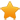Switch Editions?
CancelChannel: CodeSection,代码区,网络安全 - CodeSec
Viewing all articles

# R-plot with continuous column

0
0

This question already has an answer here:

Plotting two variables as lines using ggplot2 on the same graph 4 answers

I am willing to do some line charts in R with these data below. For now, I get an error with my code. Any help will be welcome.

data <- structure(list(names = c("AB", "BBB", "CCC"), day1 = c(2L, 4L, 14L), day2 = c(2.2, 10, 25), day3 = c(1.2, 12, 12), day4 = c(1.7, 4, 12), day5 = c(2L, 10L, 14L)), .Names = c("names", "day1", "day2", "day3", "day4", "day5"), row.names = c(NA, -3L), class = "data.frame") data #names day1 day2 day3 day4 day5 #AB 2 2.2 1.2 1.7 2 #BBB 4 10 12 4 10 #CCC 14 25 12 12 14 ggplot(data, aes(day1:day5, names, color = names)) + geom_line()

Try this:

data <- structure(list(names = c("AB", "BBB", "CCC"), day1 = c(2L, 4L, 14L), day2 = c(2.2, 10, 25), day3 = c(1.2, 12, 12), day4 = c(1.7, 4, 12), day5 = c(2L, 10L, 14L)), .Names = c("names", "day1", "day2", "day3", "day4", "day5"), row.names = c(NA, -3L), class = "data.frame") dat_m <- melt(data, id.var = "names") ggplot(df_m, aes(variable, value, colour = names, group = names)) + geom_line() This results in this plot:

Viewing all articles

### 劉克襄：被蟲咬過的紅鳳菜

More Pages to Explore .....